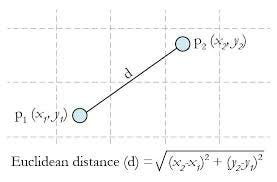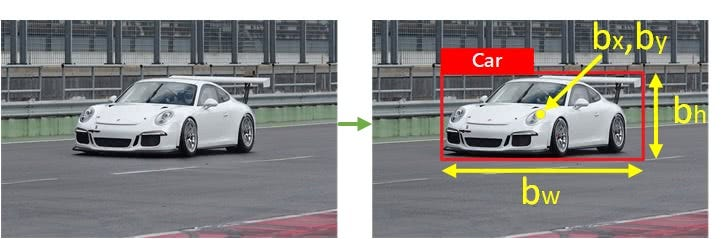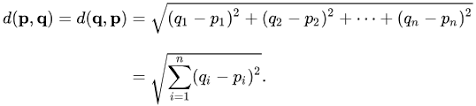distance equation

# Object Tracking — Referenced with the previous frame using Euclidean distance.

In my previous blog, I have explained what is object detection and how to detect and track multiple objects.

In this blog, I explain how to track each object from the video. I have assigned the unique label(1,2,3, etc) to each object and track it by reference with the previous frame.

#### Detection Techniques:

In yolov3 detect the objects from the image. It will return the coordinates of each object. The coordinates are x, y, width and height of each object.yolo bounding box value

#### Tracking Techniques:

I have implemented object tracking by using the previous frame. Because of the previous frame is a referenced frame. I have saved the object coordinates of a previous frame and compare it with the current frame.

Euclidean distance or Euclidean metric is the “ordinary” straight-line distance between two points in Euclidean space.

Find the distance between the current frame and the referenced frame using Euclidean distance for each object.Euclidean Distance Equation

If the distance between two object value is less than 50, this is the same object otherwise create a new label and assign it.

Numpy find distance:

``dist = numpy.linalg.norm(a-b)``

I have used pjreddie darknet for object detection. The source code is below, Just copy and paste into your darknet directory and run it.

#### Explanation:

Line 1–150: These lines of code for load yolo network and detect the object from the image.
Line 160–161: These lines mentioned for creating the reference frame object points.
Line 162: This line for assign the minimum distance between the current and previous frame.
Line 167–168: These lines mentioned for creating the current frame object points.
Line 170: The detect method used for predict the objects from the image. It has three arguments. The net refers loaded the network, meta refer label name and the final argument is detecting image.
Line 172–183: These lines calculate the bounding box from the output detection.
Line 186–196: I have implemented the tracker algorithm with Euclidean distance in those lines. Find the distance between two frames and if it is less than the minimum distance I will assign the same label name or else create a new label id.
Line 197–198: Assigned the current frame x,y points and it’s corresponding label id to the variable.
Line 201–202: Assigned the current frame points and labels to reference frame which helps to next frame.

### cons:

1. If the two persons crossing, then the one person label should be changed.
2. One frame did not detect the person, It should change the label id. Because my tracker working depends on the reference frame.

#### Conclusion:

If the model detects the object in every frame, then the tracker algorithm working perfectly.